# Finding the third point on a right triangle / circle tangent lines

The title seems simple enough, however, I can’t seem to figure out an elegant implementation.

Context: I’m trying to make a custom parametric function with a minimum turning radius at the ends.

To do this, I am trying to find the coordinates of point C here:

And ideally, I’d like to get both possible points for C: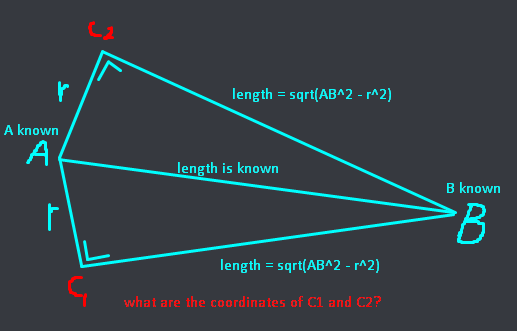My current implementation looked like this:

``````local XC = XB - math.sin(math.rad(90) - (math.asin(AC/AB) - math.asin((YB - YA) / AB))) * BC
local YC = YB + math.cos(math.rad(90) - (math.asin(AC/AB) - math.asin((YB - YA) / AB))) * BC
``````

This, however, only finds one of the points and only if point B has a higher ‘Y’ coordinate than A, otherwise it pretty much breaks.
Would there be a cleaner solution for this?
I’ve heard someone suggest vector maths, would that be worth looking into?

3 Likes

I imagine in 3D space there is an infinite amount of coordinates that can be generated.

I think you can imagine it as an ice cream cone cone shape hence the current formula breaks according to this condition:

I imagine you would need to define an additional plane normal vector like vector (0,1,0) in order to get a rotation axis.

Then calculate angle <BAC1 using cosine formula

Then rotate vector A to B around the plane axis vector around an axis (0,1,0) clockwise and counterclockwise by this angle to get the direction vector of r1 and r2 then you can construct the vector path towards the coordinates A +r1.

I believe there’s a better solution but it’s night time for me hopefully someone else has a much better solution. Like converting 3d to 2d and vice versa usually done by using math planes to do 2d to 3d defining 2d coordinates in 3d space.

1 Like

I forgot to mention, but you can think about this problem as if exists only in the horizontal plane.
The comment about the ‘Y’ coordinate of B being lower than A referred to the Roblox ‘Z’ coordinate.

All the Y-values mentioned in my original post actually refer to Roblox Z coordinate. So there are in fact only 2 possible solutions

You’d have to find the tangent that works for both the circles. This thread might be a bit of help:

I’m not too sure about how you do the calculations to get both point `C`s but a general idea I have in mind is that both the triangles are right triangles. The bases of both the right triangles are perpendicular and coincident:

Basically the idea is to find a point on the perimeter of circle (`C`) that creates a right triangle with the points `A` and `B`.

You already have the hypotenuse and one leg. You just have to find the length of the last leg (line `BC`) and the angle of `ACB`.

This is just a theory and I might not be correct.

1 Like

This is correct. I do have the length of CB. It’s simply sqrt(AB^2 - AC^2) (pythagoras).

That’s also why the problem can be simplified down to a simple triangle ABC only.

I’ll take a look at the article you’ve linked, thanks for that.

I’m kinda confused what you’re trying to achieve here. Do you mean something like this?

https://gyazo.com/b04e44a8ca03305a075b3d7bab07db16

No, I’m trying to find the transverse common tangents: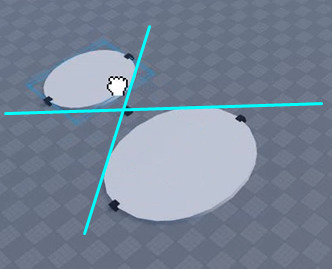To do that, I have to find the third point of the triangle I’ve posted earlier. (However, if you have a completely different solution for this, I’m all ears.)

This is an instance where it pays off to know your geometric trig definitions

Secant is the distance from the origin of the intersection of a line perpendicular to the tangent line with the x axis

You can probably already see why this is useful

You can very easily relate half the distance between the two circles apart with the angle of the tangent point that intersects with this point

Using this formula: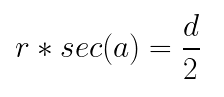(Where r is the radius and d is the distance between the two circles)

You can find the angle between the four points, the only thing you really need is just: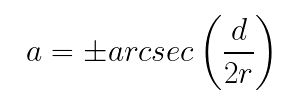Roblox of course doesnt have arcsecant so good thing that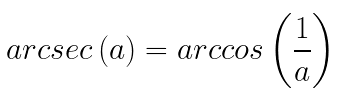Therefore: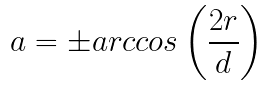This can be easily transferred to the circle through a basic cframe transformation to rotate it into reference along with some cos a, sin a to get the point around the circle with that angle

If you dont want to use the cframe of the circle for whatever reason you can also just do:

``````position1 = A + (B-A).Unit * r * math.cos(a) + (B-A).Unit:Cross(Vector3.new(0,1,0)) * r * math.sin(a)
position2 = A + (B-A).Unit * math.cos(-a) + (B-A).Unit:Cross(Vector3.new(0,1,0)) * r * math.sin(-a)
position3 = B + (B-A).Unit * r * -math.cos(a) + (B-A).Unit:Cross(Vector3.new(0,1,0)) * r * math.sin(a)
position4 = B + (B-A).Unit * r * -math.cos(-a) + (B-A).Unit:Cross(Vector3.new(0,1,0)) * r * math.sin(-a)
``````

(This is super ugly though and not all that simple)
You dont necessarily have to use Vector3.new(0,1,0) and it might be better to just use the -rightvector of the cylinder, depending on what youre doing but whatever works

6 Likes

Thanks for putting the time and effort into this incredible solution.
Exactly what I needed, super in-depth too!

1 Like

Well uhhh it might be too late, but you can do this by just doing algebra. However, there does come a point where you do need to use trig, and it’s impossible to avoid using it. Regardless, this is the method.

The circles in the XY Cartesian plane look something like this: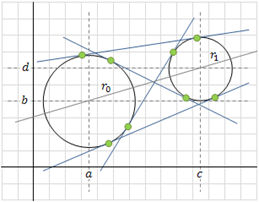We know that the equations of the circles are:

(x − a)² + (y − b)² = r₀²
(x − c)² + (y − d)² = r₁²

We also know that, in general, two circles can have up to 4 common tangent lines. These are the outer tangent lines, and the inner tangent lines. We will begin by finding the outer tangent lines. To find the outer tangent lines, we can refer to this image: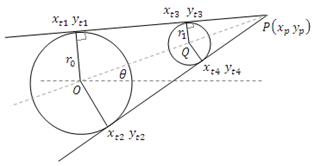We want to calculate the intersection of these two outer tangents, which is at the point P(xₚ, yₚ). To calculate these coordinates, we can use the equations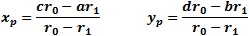Where r₀ > r₁. To get the point P(xₚ, yₚ). Now that we have point P, we can continue to find the outer points of both circles. Using the equations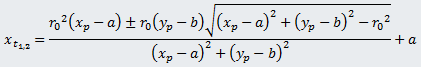and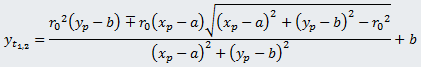We can get the points (xₜ₁, yₜ₁) and (xₜ₂, yₜ₂), which are the outer points on circle 1. We still need to know the outer points for circle 2,so we can use similar equations to solve for the outer tangent points on circle 2.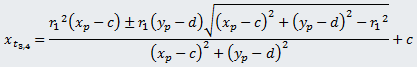and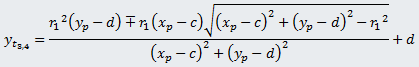The outer points for circle 2 are now coordinates (xₜ₃, yₜ₃) and (xₜ₄, yₜ₄). We now have the outer tangent points, but we still need to find the inner tangent points (in this case, the ones you are looking for). To find these, we can use a similar method.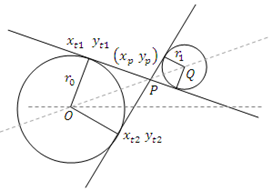In the image above, we can figure out the intersection point of the two inner tangent lines by using the two equations below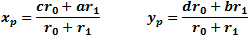Now, we can go ahead and find the inner tangent points of both circles. For circle 1, the tangent points will be given with these equations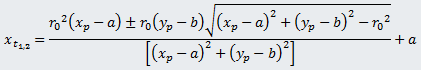and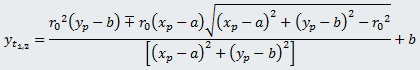Next, we can find the inner tangent points of the second circle using the equations below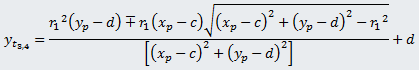and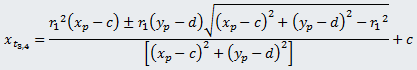We now have all the tangent points that we can find in these circles. However, there are some things that can go wrong. Wherever we see square roots, there are some solutions that could possibly be imaginary. This table below can show the possible outcomes. For reasons useful for the below table, we will refer to the distance between the centers of both circles as D.

Graph 1: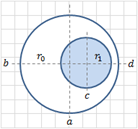This type of graph contains 0 common tangent lines. In general, when D < |r₀ - r₁|, the circles will not have any tangent lines in common.

Graph 2: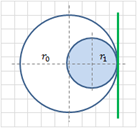This type of graph contains 1 common tangent line. In general, when D = |r₀ - r₁|, there will be one common tangent point. This is located at the edge of where both circles meet.

Graph 3: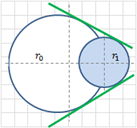This type of graph contains 2 common tangent lines. In general, when |r0 − r1 | < D < r0 + r1, the circles will share 2 tangent lines. This happens when one circle is in between the other.

Graph 4: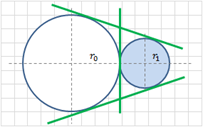This type of graph contains 3 common tangent lines. In general, when D = r0 + r1, the circles will share 3 tangent lines. This happens when one circle is at the very edge of the other.

Graph 5: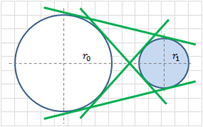This type of graph contains 4 common tangent lines. In general, when D > r0 + r1, the circles will share 4 tangent lines. This happens when the circles have space in between each other. However, as D approaches infinity, the number of tangent points shared between the two circles will become 2.

Although this method is almost full proof, it does come with some limitations. For example, when the circles happen to have the same radius, we’re unable to get the tangent points on the outsides of the circle. This is because the outer tangent lines never intersect, so we can’t find the outer lines. However, this method will always give the inner tangent lines, regardless of whether the circles are the same size or not.

In code, this looks something like this:

``````local circles = {
workspace.C1;
workspace.C2;
}

local function createPoint(name, thickness)
if thickness == nil then
thickness = 1
end
local p = Instance.new("Part", workspace)
p.Locked = true
p.Anchored = true
p.CanCollide = false
p.Size = Vector3.new(1, 1, 1) * thickness
p.Color = Color3.fromRGB(0, 0, 0)
p.Name = name
return p
end

local c1Point = createPoint("C1", 1.5)
local c2Point = createPoint("C2", 1.5)
local outerPoint1 = createPoint("OuterPoint1", 1.5)
local outerPoint2 = createPoint("OuterPoint2", 1.5)
local outerPoint3 = createPoint("OuterPoint3", 1.5)
local outerPoint4 = createPoint("OuterPoint4", 1.5)
local innerPoint1 = createPoint("InnerPoint1", 1.5)
local innerPoint2 = createPoint("InnerPoint2", 1.5)
local innerPoint3 = createPoint("InnerPoint3", 1.5)
local innerPoint4 = createPoint("InnerPoint4", 1.5)
function update()
-- turn into a 2d problem where we focus only on the xz coordinate system  (we will reference it as x and y in the code)
local c1, c2 = Vector2.new(circles.Position.X, circles.Position.Z), Vector2.new(circles.Position.X, circles.Position.Z)
local radii = {c1 = 0.5 * math.min(circles.Size.Y, circles.Size.Z), c2 = 0.5 * math.min(circles.Size.Y, circles.Size.Z)}
local a, b, c, d = r0 == radii.c1 and c1.X or c2.X, r0 == radii.c1 and c1.Y or c2.Y, r1 == radii.c1 and c1.X or c2.X, r1 == radii.c1 and c1.Y or c2.Y
local D = math.sqrt((c - a)^2 + (d - b)^2)

-- saved points
local points = {
circle1 = {outerPoints = {}, innerPoints = {}},
circle2 = {outerPoints = {}, innerPoints = {}}
}

-- intersection points for outer tangents
local x_p = (c * r0 - a * r1) / (r0 - r1)
local y_p = (d * r0 - b * r1) / (r0 - r1)

-- find outer tangents in circle 1
local x_t1 = ((r0^2 * (x_p - a) + r0 * (y_p - b) * math.sqrt((x_p - a)^2 + (y_p - b)^2 - r0^2)) / ((x_p - a)^2 + (y_p - b)^2)) + a
local x_t2 = ((r0^2 * (x_p - a) - r0 * (y_p - b) * math.sqrt((x_p - a)^2 + (y_p - b)^2 - r0^2)) / ((x_p - a)^2 + (y_p - b)^2)) + a
local y_t1 = ((r0^2 * (y_p - b) - r0 * (x_p - a) * math.sqrt((x_p - a)^2 + (y_p - b)^2 - r0^2)) / ((x_p - a)^2 + (y_p - b)^2)) + b
local y_t2 = ((r0^2 * (y_p - b) + r0 * (x_p - a) * math.sqrt((x_p - a)^2 + (y_p - b)^2 - r0^2)) / ((x_p - a)^2 + (y_p - b)^2)) + b
table.insert(points.circle1.outerPoints, Vector2.new(x_t1, y_t1))
table.insert(points.circle1.outerPoints, Vector2.new(x_t2, y_t2))

-- find outer tangents in circle 2
local x_t3 = ((r1^2 * (x_p - c) + r1 * (y_p - d) * math.sqrt((x_p - c)^2 + (y_p - d)^2 - r1^2)) / ((x_p - c)^2 + (y_p - d)^2)) + c
local x_t4 = ((r1^2 * (x_p - c) - r1 * (y_p - d) * math.sqrt((x_p - c)^2 + (y_p - d)^2 - r1^2)) / ((x_p - c)^2 + (y_p - d)^2)) + c
local y_t3 = ((r1^2 * (y_p - d) - r1 * (x_p - c) * math.sqrt((x_p - c)^2 + (y_p - d)^2 - r1^2)) / ((x_p - c)^2 + (y_p - d)^2)) + d
local y_t4 = ((r1^2 * (y_p - d) + r1 * (x_p - c) * math.sqrt((x_p - c)^2 + (y_p - d)^2 - r1^2)) / ((x_p - c)^2 + (y_p - d)^2)) + d
table.insert(points.circle2.outerPoints, Vector2.new(x_t3, y_t3))
table.insert(points.circle2.outerPoints, Vector2.new(x_t4, y_t4))

-- intersection points for inner tangents
local x_p = (c * r0 + a * r1) / (r0 + r1)
local y_p = (d * r0 + b * r1) / (r0 + r1)

-- find inner tangents in circle 1
local x_t1 = ((r0^2 * (x_p - a) + r0 * (y_p - b) * math.sqrt((x_p - a)^2 + (y_p - b)^2 - r0^2)) / ((x_p - a)^2 + (y_p - b)^2)) + a
local x_t2 = ((r0^2 * (x_p - a) - r0 * (y_p - b) * math.sqrt((x_p - a)^2 + (y_p - b)^2 - r0^2)) / ((x_p - a)^2 + (y_p - b)^2)) + a
local y_t1 = ((r0^2 * (y_p - b) - r0 * (x_p - a) * math.sqrt((x_p - a)^2 + (y_p - b)^2 - r0^2)) / ((x_p - a)^2 + (y_p - b)^2)) + b
local y_t2 = ((r0^2 * (y_p - b) + r0 * (x_p - a) * math.sqrt((x_p - a)^2 + (y_p - b)^2 - r0^2)) / ((x_p - a)^2 + (y_p - b)^2)) + b
table.insert(points.circle1.innerPoints, Vector2.new(x_t1, y_t1))
table.insert(points.circle1.innerPoints, Vector2.new(x_t2, y_t2))

-- find inner tangents in circle 2
local x_t3 = ((r1^2 * (x_p - c) + r1 * (y_p - d) * math.sqrt((x_p - c)^2 + (y_p - d)^2 - r1^2)) / ((x_p - c)^2 + (y_p - d)^2)) + c
local x_t4 = ((r1^2 * (x_p - c) - r1 * (y_p - d) * math.sqrt((x_p - c)^2 + (y_p - d)^2 - r1^2)) / ((x_p - c)^2 + (y_p - d)^2)) + c
local y_t3 = ((r1^2 * (y_p - d) - r1 * (x_p - c) * math.sqrt((x_p - c)^2 + (y_p - d)^2 - r1^2)) / ((x_p - c)^2 + (y_p - d)^2)) + d
local y_t4 = ((r1^2 * (y_p - d) + r1 * (x_p - c) * math.sqrt((x_p - c)^2 + (y_p - d)^2 - r1^2)) / ((x_p - c)^2 + (y_p - d)^2)) + d
table.insert(points.circle2.innerPoints, Vector2.new(x_t3, y_t3))
table.insert(points.circle2.innerPoints, Vector2.new(x_t4, y_t4))

-- move outer points
outerPoint1.CFrame = CFrame.new(points.circle1.outerPoints.X, 0.5, points.circle1.outerPoints.Y)
outerPoint2.CFrame = CFrame.new(points.circle1.outerPoints.X, 0.5, points.circle1.outerPoints.Y)
outerPoint3.CFrame = CFrame.new(points.circle2.outerPoints.X, 0.5, points.circle2.outerPoints.Y)
outerPoint4.CFrame = CFrame.new(points.circle2.outerPoints.X, 0.5, points.circle2.outerPoints.Y)

-- move inner points
innerPoint1.CFrame = CFrame.new(points.circle1.innerPoints.X, 0.5, points.circle1.innerPoints.Y)
innerPoint2.CFrame = CFrame.new(points.circle1.innerPoints.X, 0.5, points.circle1.innerPoints.Y)
innerPoint3.CFrame = CFrame.new(points.circle2.innerPoints.X, 0.5, points.circle2.innerPoints.Y)
innerPoint4.CFrame = CFrame.new(points.circle2.innerPoints.X, 0.5, points.circle2.innerPoints.Y)
end
for _, circle in pairs(circles) do
circle.Changed:Connect(function(prop)
if prop == "Position" or prop == "Size" then
update()
end
end)
end
update()
``````

The outcome looks like this:

Hopefully this gives insight in another method that doesn’t involve trig!

3 Likes

Thanks for the super in-depth working out. It works almost perfectly.
Do you know what I’d have to change to make it also work for circles with the same radius?
This isn’t an edge-case for me, all the circles in my project will be of the same radius.

I guess I could add 0.0001 to the radius of one of them to make it work, but I feel like this is kind of a hacky solution?

The x offset from the center of both circles going directly towards the center point I think can just be defined algebraically as:

``````x=2r^2/d
``````

Where both radii are the same

You can then get the positions by plugging in the circle formula (make sure you plus minus the y value to get both sides) or just using trig if you want and using a similar method to my previous post (either CFrame or vector stuff)

You can derive this formula by saying

``````f(x)=sqrt(r^2-x^2)
f’(x)=-x/sqrt(r^2-x^2) --graph of the slope of f(x) at x
--Use point slope to find a value of x such that the slope of that point to (d/2,0) == the slope of the circle at that point, x
f’(x)=(f(x)-0)/(x-d/2)
--Solve and you get that equation, x=2r^2/d
``````

I guess this is even simpler than arcsec(d/2r) in a certain sense

1 Like

The method will always give you the inner tangent lines, so you don’t need to worry about the inner lines. But the outer ones, they have to be calculated using trigonometry.

Let’s say we have two circles with radius r. They would look like this on the XY cartesian plane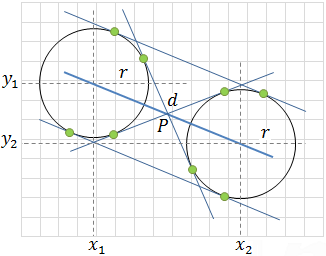and would have the equations

(x − x1) + (y − y1) = r2
(x − x2) + (y − y2) = r2

Now ignoring the inner tangent lines, we’re only looking at the outer tangent lines. We can solve for the outer tangent lines by looking at this image as an example.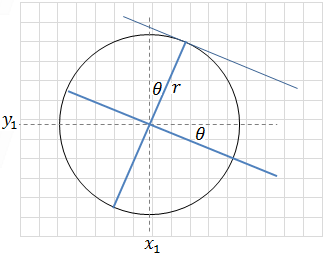You can see that the line from the midpoint of circle 1 to circle 2 is rotated at an angle theta, and is translated to the edge of the circle by a distance of r. We can get the slope of the angle theta by using the equations: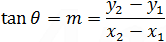and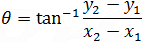Note: use atan2 in coding to get rid of x2-x1 = 0 error

Now that we have theta, we can solve for the equations of the points outside, we can solve for the tangent points outside of both of the circles. We can use the equations: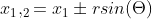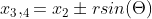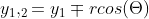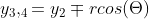We should now have 4 points constructed as (x1, y1), (x2, y2), (x3, y3), and (x4, y4). These are the points we can use to construct the outer tangent lines of the circles.

In code, it looks like this:

``````local circles = {
workspace.C1;
workspace.C2;
}

local function createPoint(name, thickness)
if thickness == nil then
thickness = 1
end
local p = Instance.new("Part", workspace)
p.Locked = true
p.Anchored = true
p.CanCollide = false
p.Size = Vector3.new(1, 1, 1) * thickness
p.Color = Color3.fromRGB(0, 0, 0)
p.Name = name
return p
end

local c1Point = createPoint("C1", 1.5)
local c2Point = createPoint("C2", 1.5)
local outerPoint1 = createPoint("OuterPoint1", 1.5)
local outerPoint2 = createPoint("OuterPoint2", 1.5)
local outerPoint3 = createPoint("OuterPoint3", 1.5)
local outerPoint4 = createPoint("OuterPoint4", 1.5)
local innerPoint1 = createPoint("InnerPoint1", 1.5)
local innerPoint2 = createPoint("InnerPoint2", 1.5)
local innerPoint3 = createPoint("InnerPoint3", 1.5)
local innerPoint4 = createPoint("InnerPoint4", 1.5)
function update()
-- turn into a 2d problem where we focus only on the xz coordinate system  (we will reference it as x and y in the code)
local c1, c2 = Vector2.new(circles.Position.X, circles.Position.Z), Vector2.new(circles.Position.X, circles.Position.Z)
local radii = {c1 = 0.5 * math.min(circles.Size.Y, circles.Size.Z), c2 = 0.5 * math.min(circles.Size.Y, circles.Size.Z)}
local a, b, c, d

-- saved points
local points = {
circle1 = {outerPoints = {}, innerPoints = {}},
circle2 = {outerPoints = {}, innerPoints = {}}
}

-- check if r0 and r1 are equal radii
if r0 == r1 then

-- label a, b, c, d
a, b, c, d = c1.X, c1.Y, c2.X, c2.Y

-- label radius as one variable r
local r = r0

-- find angle theta (rotation of the line going through midpoints)
local x_1, x_2 = a, c
local y_1, y_2 = b, d
local theta = math.atan2((y_2 - y_1), (x_2 - x_1))

-- get points at outer edges of circle 1
local px_1 = x_1 + r * math.sin(theta)
local px_2 = x_1 - r * math.sin(theta)
local py_1 = y_1 - r * math.cos(theta)
local py_2 = y_1 + r * math.cos(theta)
table.insert(points.circle1.outerPoints, Vector2.new(px_1, py_1))
table.insert(points.circle1.outerPoints, Vector2.new(px_2, py_2))

-- get points at outer edges of circle 2
local px_3 = x_2 + r * math.sin(theta)
local px_4 = x_2 - r * math.sin(theta)
local py_3 = y_2 - r * math.cos(theta)
local py_4 = y_2 + r * math.cos(theta)
table.insert(points.circle2.outerPoints, Vector2.new(px_3, py_3))
table.insert(points.circle2.outerPoints, Vector2.new(px_4, py_4))
else

-- label a, b, c, d
a, b, c, d = r0 == radii.c1 and c1.X or c2.X, r0 == radii.c1 and c1.Y or c2.Y, r1 == radii.c1 and c1.X or c2.X, r1 == radii.c1 and c1.Y or c2.Y

-- intersection points for outer tangents
local x_p = (c * r0 - a * r1) / (r0 - r1)
local y_p = (d * r0 - b * r1) / (r0 - r1)

-- find outer tangents in circle 1
local x_t1 = ((r0^2 * (x_p - a) + r0 * (y_p - b) * math.sqrt((x_p - a)^2 + (y_p - b)^2 - r0^2)) / ((x_p - a)^2 + (y_p - b)^2)) + a
local x_t2 = ((r0^2 * (x_p - a) - r0 * (y_p - b) * math.sqrt((x_p - a)^2 + (y_p - b)^2 - r0^2)) / ((x_p - a)^2 + (y_p - b)^2)) + a
local y_t1 = ((r0^2 * (y_p - b) - r0 * (x_p - a) * math.sqrt((x_p - a)^2 + (y_p - b)^2 - r0^2)) / ((x_p - a)^2 + (y_p - b)^2)) + b
local y_t2 = ((r0^2 * (y_p - b) + r0 * (x_p - a) * math.sqrt((x_p - a)^2 + (y_p - b)^2 - r0^2)) / ((x_p - a)^2 + (y_p - b)^2)) + b
table.insert(points.circle1.outerPoints, Vector2.new(x_t1, y_t1))
table.insert(points.circle1.outerPoints, Vector2.new(x_t2, y_t2))

-- find outer tangents in circle 2
local x_t3 = ((r1^2 * (x_p - c) + r1 * (y_p - d) * math.sqrt((x_p - c)^2 + (y_p - d)^2 - r1^2)) / ((x_p - c)^2 + (y_p - d)^2)) + c
local x_t4 = ((r1^2 * (x_p - c) - r1 * (y_p - d) * math.sqrt((x_p - c)^2 + (y_p - d)^2 - r1^2)) / ((x_p - c)^2 + (y_p - d)^2)) + c
local y_t3 = ((r1^2 * (y_p - d) - r1 * (x_p - c) * math.sqrt((x_p - c)^2 + (y_p - d)^2 - r1^2)) / ((x_p - c)^2 + (y_p - d)^2)) + d
local y_t4 = ((r1^2 * (y_p - d) + r1 * (x_p - c) * math.sqrt((x_p - c)^2 + (y_p - d)^2 - r1^2)) / ((x_p - c)^2 + (y_p - d)^2)) + d
table.insert(points.circle2.outerPoints, Vector2.new(x_t3, y_t3))
table.insert(points.circle2.outerPoints, Vector2.new(x_t4, y_t4))
end

-- intersection points for inner tangents
local x_p = (c * r0 + a * r1) / (r0 + r1)
local y_p = (d * r0 + b * r1) / (r0 + r1)

-- find inner tangents in circle 1
local x_t1 = ((r0^2 * (x_p - a) + r0 * (y_p - b) * math.sqrt((x_p - a)^2 + (y_p - b)^2 - r0^2)) / ((x_p - a)^2 + (y_p - b)^2)) + a
local x_t2 = ((r0^2 * (x_p - a) - r0 * (y_p - b) * math.sqrt((x_p - a)^2 + (y_p - b)^2 - r0^2)) / ((x_p - a)^2 + (y_p - b)^2)) + a
local y_t1 = ((r0^2 * (y_p - b) - r0 * (x_p - a) * math.sqrt((x_p - a)^2 + (y_p - b)^2 - r0^2)) / ((x_p - a)^2 + (y_p - b)^2)) + b
local y_t2 = ((r0^2 * (y_p - b) + r0 * (x_p - a) * math.sqrt((x_p - a)^2 + (y_p - b)^2 - r0^2)) / ((x_p - a)^2 + (y_p - b)^2)) + b
table.insert(points.circle1.innerPoints, Vector2.new(x_t1, y_t1))
table.insert(points.circle1.innerPoints, Vector2.new(x_t2, y_t2))

-- find inner tangents in circle 2
local x_t3 = ((r1^2 * (x_p - c) + r1 * (y_p - d) * math.sqrt((x_p - c)^2 + (y_p - d)^2 - r1^2)) / ((x_p - c)^2 + (y_p - d)^2)) + c
local x_t4 = ((r1^2 * (x_p - c) - r1 * (y_p - d) * math.sqrt((x_p - c)^2 + (y_p - d)^2 - r1^2)) / ((x_p - c)^2 + (y_p - d)^2)) + c
local y_t3 = ((r1^2 * (y_p - d) - r1 * (x_p - c) * math.sqrt((x_p - c)^2 + (y_p - d)^2 - r1^2)) / ((x_p - c)^2 + (y_p - d)^2)) + d
local y_t4 = ((r1^2 * (y_p - d) + r1 * (x_p - c) * math.sqrt((x_p - c)^2 + (y_p - d)^2 - r1^2)) / ((x_p - c)^2 + (y_p - d)^2)) + d
table.insert(points.circle2.innerPoints, Vector2.new(x_t3, y_t3))
table.insert(points.circle2.innerPoints, Vector2.new(x_t4, y_t4))

-- move outer points
outerPoint1.CFrame = CFrame.new(points.circle1.outerPoints.X, 0.5, points.circle1.outerPoints.Y)
outerPoint2.CFrame = CFrame.new(points.circle1.outerPoints.X, 0.5, points.circle1.outerPoints.Y)
outerPoint3.CFrame = CFrame.new(points.circle2.outerPoints.X, 0.5, points.circle2.outerPoints.Y)
outerPoint4.CFrame = CFrame.new(points.circle2.outerPoints.X, 0.5, points.circle2.outerPoints.Y)

-- move inner points
innerPoint1.CFrame = CFrame.new(points.circle1.innerPoints.X, 0.5, points.circle1.innerPoints.Y)
innerPoint2.CFrame = CFrame.new(points.circle1.innerPoints.X, 0.5, points.circle1.innerPoints.Y)
innerPoint3.CFrame = CFrame.new(points.circle2.innerPoints.X, 0.5, points.circle2.innerPoints.Y)
innerPoint4.CFrame = CFrame.new(points.circle2.innerPoints.X, 0.5, points.circle2.innerPoints.Y)
end
for _, circle in pairs(circles) do
circle.Changed:Connect(function(prop)
if prop == "Position" or prop == "Size" then
update()
end
end)
end
update()
``````

Then we run the simulation:

Sorry I take so long to reply, I ended up finishing my work at school and I had enough time to write this answer lol

2 Likes